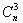Competitions

# Circum Triangle

You will be given n distinct points on the boundary of a circle whose center is at the origin. As the points are on the same circle no three of them are collinear, so any three of them creates a valid triangle. Your job is to find the summation of areas of thesetriangles.

Input

Input file contains at most 16 sets of inputs. Each set starts with two integers n (0n500) and r (0 < r100). Here n is the number of points and r is the radius of the circle. You can assume that the center of the circle is always at the origin. This line is followed by n lines each of which contains a floating-point number θ (0.0θ < 360.00) which actually denotes the angle in degree the designated point creates with respect to the origin with x-axis. So for example if θ is 30.00 degree then the Cartesian coordinate of the intended point is (rcos(30.00°), rsin(30.00°)). The last line contains n = r = 0 and is not processed.

Output

For each test case produce one line of output. This line contains an integer number which is the total area (rounded to nearest integer) of all possible triangles formed by the given n points. The judge data will be such that small precision errors will not cause the output to be different.

Time limit 1 second
Memory limit 64 MiB
Input example #1
5 10
10.00
100.00
300.00
310.00
320.00
3 20
10.00
100.00
300.00
0 0

Output example #1
286
320